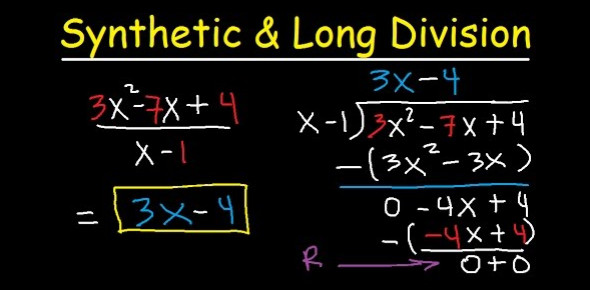# Synthetic And Long Division Test! Trivia Quiz

10 Questions | Total Attempts: 137Settings.

• 1.
• A.

X-4

• B.

2x+4

• C.

X+4

• D.

2x-4

• 2.
• A.

-x+3

• B.

5x-4

• C.

-5x+4

• D.

5x+3

• 3.
• A.

X^2-3x+1

• B.

X^2+3x-1

• C.

X^2-5x+4

• D.

X^2+5x-4

• 4.
Synthetic division can always be used when dividing polynomials.
• A.

True

• B.

False

• 5.
When dividing a polynomial of degree 6 by a polynomial of degree 2, the quotient will be a polynomial of degree 3.
• A.

True

• B.

False

• 6.
• A.

3x^2-5

• B.

-3x+2

• C.

-3x+2x-5

• D.

3x^2-2x+5

• 7.
• A.

X^2+1

• B.

X^2-1

• C.

X^2-x-1

• D.

X^2+x+1

• 8.
• A.

-x^3+6x^2-3x

• B.

2x^3-6x^2+3

• C.

X^3-6x

• D.

X^3-6x^2+3x

• E.

None of the above

• 9.
When dividing   by    ,  the constant in the answer is _______ .
• 10.
The ________________ theorem allows you to evaluate a function at a given value of x by simply using synthetic division and using the given value of x as the number used as the divisor in the synthetic division.
Related TopicsBack to top